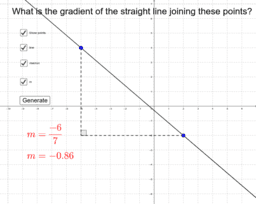Mr Hardman

•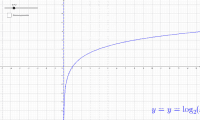Logarithmic graphs (OneNote)

Activity

Mr Hardman

•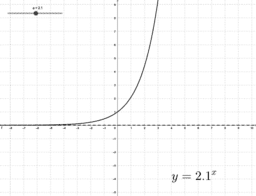Exponential graphs (OneNote)

Activity

Mr Hardman

•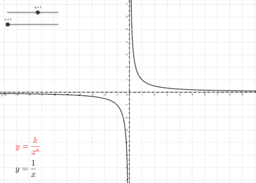Graphs of reciprocal functions

Activity

Mr Hardman

•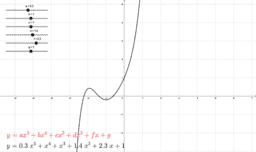Graphs of polynomials (OneNote)

Activity

Mr Hardman

•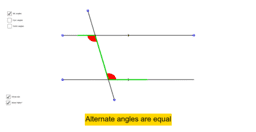Angles in parallel lines (OneNote)

Activity

Mr Hardman

•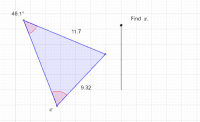Sine rule - missing angle

Activity

Mr Hardman

•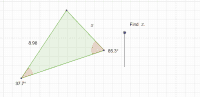Sine rule - missing side

Activity

Mr Hardman

•Cosine rule - missing side

Activity

Mr Hardman

•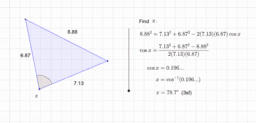Cosine rule - missing angle

Activity

Mr Hardman

•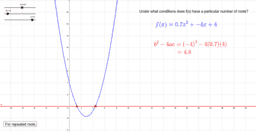Discriminant (OneNote)

Activity

Mr Hardman

•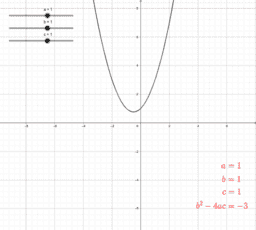Activity

Mr Hardman

•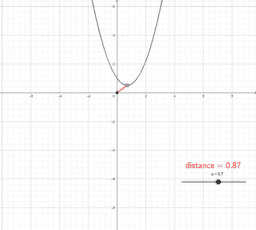Activity

Mr Hardman

•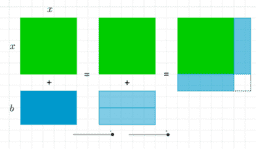Completing the square (OneNote)

Activity

Mr Hardman

•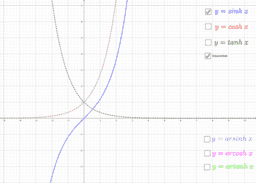Hyperbolic functions graphs (OneNote)

Activity

Mr Hardman

•Hyperbolic functions (OneNote)

Activity

Mr Hardman

•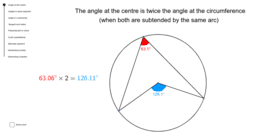Circle theorems with proofs (OneNote)

Activity

Mr Hardman

•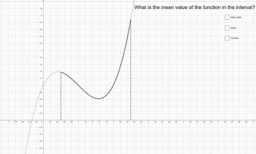Mean value of a function (OneNote)

Activity

Mr Hardman

•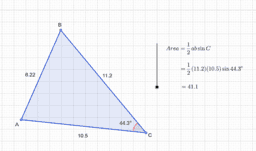Area of a triangle

Activity

Mr Hardman

•Sine rule ambiguous case

Activity

Mr Hardman

•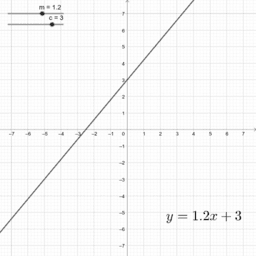Straight lines 1

Activity

Mr Hardman

•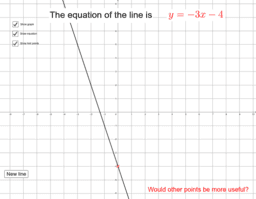y = mx + c

Activity

Mr Hardman

•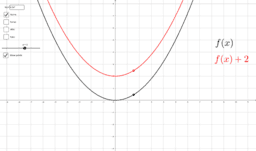Transformations of functions

Activity

Mr Hardman

•Straight line

Activity

Mr Hardman

•